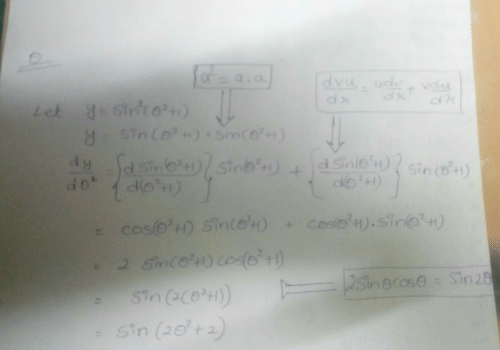Differentiate sin2(θ2 + 1) with respect ...

### Related TestDifferentiate sin22 + 1) with respect to θ2
• a)
sin(2θ2 + 1)
• b)
cos(2θ2 + 2)
• c)
sin(2θ2 + 2)
• d)
cos(2θ2 + 1)Sonålí Raåz
First u just differentiate sin^2(ø^2+1) with respect to sin(ø^2+1) so we get 2sin(ø^2+1)..Then again differentiate sin(ø^2+1) with respect to (ø^2+1) so we get cos(ø^2+1). and now lastly we differentiate (ø^2+1) with respect to ø^2 so we get 1 ..... and lastly the result is 2sin(ø^2+1)×cos(ø^2+1)×1 = sin2(ø^2+1) = sin(2ø^2+2)..... Here , ø= theta ....

 1 Crore+ students have signed up on EduRev. Have you?Saksham Nagar
Differentiate sin2(θ2 + 1) with respect toθ2a)sin(2θ2 + 1)b)cos(2θ2 + 2)c)sin(2θ2 + 2)d)cos(2θ2 + 1)Correct answer is option 'C'. Can you explain this answer?View courses related to this question Explore JEE courses
 Explore JEE coursesView courses related to this question• ### A line makes the same angle with each of the x - axis and z - axis. It mak... more(Scan QR code)